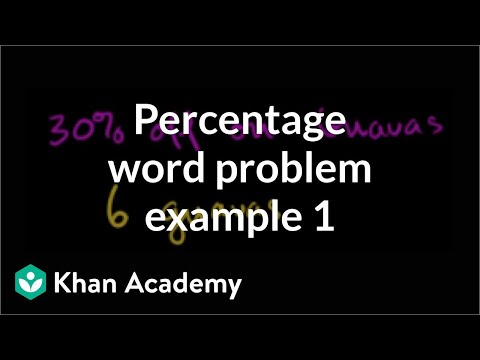Video

# Two-step equation word problem: oranges (Full video)

Description: Learn how to solve a word problem by writing an equation to model the situation. In this video, we use the linear equation 210(t - 5) = 41,790. Created by Sal Khan. MacDonald had a farm with a certain number of orange trees. So this is the number of trees initially. Well, he started with t, and he had to cut down 5, so he's going to have t minus 5 trees now.

### Other videos you might be interested in### Percent word problem: guavas (Full video)